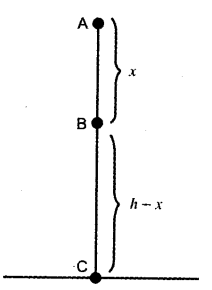# (i) State the law of conservation of energy. (ii) Show that the energy of a freely falling, body is conserved

(i) It states that for a body falling freely the total mechanical energy remains conserved.
(ii) Suppose a ball of mass ‘m’ falls under the effect of gravity as shown in figure.
Let us find the kinetic and the potential energy of the ball at various points of its free fall. Let the ball fall from point A at a height h above the surface of the earth.At Point A: At point A, the ball is stationary; therefore, its velocity is zero.
Therefore, kinetic energy, T = 0 and potential energy, U = mgh
Hence, total mechanical energy at point A is
E = T + U = 0 + mgh = mgh … (i)
At Point B : Suppose the ball covers a distance x when it moves from A to B. Let v be the velocity of the ball point B. Then by the equation of motion v^2-u^2 = 2aS, we have
v^2 - 0 = 2gx or v^2 = 2gx Therefore,
Kinetic energy, T = 1/2 mv^2 = 1/2 x m x (2gx)
= mgx
And Potential energy, U = mg (h - x)
Hence, total energy at point B is
E = T + U = mgx + mg(h-x) = mgh …(ii)
At Point C : Suppose the ball covers a distance h when it moves from A to C. Let V be the velocity of the ball at point C just before it touches the ground. Then by the equation of motion v^2 - u^2 = 2aS, we have V^2 - 0 = 2gh or V^2 = 2gh.
Therefore,
Kinetic energy,
T = 1/2 mV^2 = 1/2 x m x (2gh) = mgh
and Potential energy, U = 0
Hence, total energy at point E = T + U
= mgh + 0 = mgh … (iii)
Thus, it is clear from equations (i), (ii) and (iii), that the total mechanical energy of a freely falling ball remains constant.